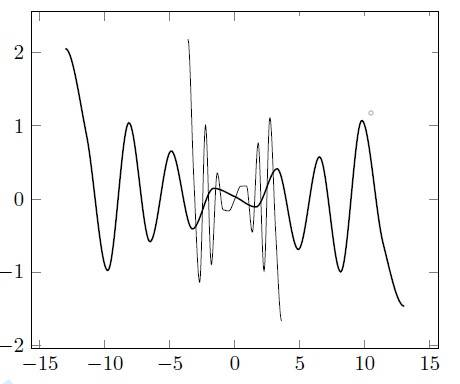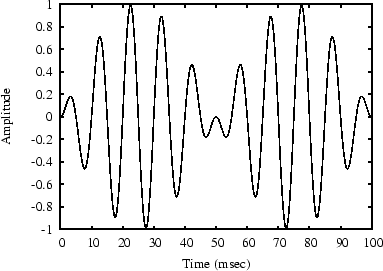# Functional representation of the oscillating graph

• yilmaz
Actually since the x-axis (the domain of the function) is also subject to evolution, the result may come in different shapes. But you can take only one of them as reference.

#### yilmaz

Homework Statement
this is actually not a homework
Relevant Equations
tsin(ct)
Hi;

This is in fact not a homework question, but it rather comes out of personal curiosity.
If you look at the graph of the two functions in the image attached, what is the simplest functional representation for such a symmetrical pattern?Welcome to PF.Can you tell us more about where you got these plots? What do they represent? Can you give a link to the website or paper where you found them? Thanks.

•yilmaz
They look like ##x \sin(\omega x)##, which I see you have indicated already. What is wrong with that?

haruspex said:
They look like ##x \sin(\omega x)##, which I see you have indicated already. What is wrong with that?
Hi @haruspex,

It actually works, but only for positive x. For negative x, I want to see it mirrored, and in addition I would like to avoid using abs or conditionals in the function.

berkeman said:
Welcome to PF.Can you tell us more about where you got these plots? What do they represent? Can you give a link to the website or paper where you found them? Thanks.
hi berkeman,

It’s actually my plot, so there’s no link. It’s the result of an evolutionary algorithm for a specific problem , where I consistently get such graphs. Since I’m not a physicist, I wanted to consult if there is any simple function representation that fits the shape.

•yilmaz
yilmaz said:
It’s actually my plot, so there’s no link. It’s the result of an evolutionary algorithm for a specific problem , where I consistently get such graphs.
What is the difference between the two plots? Did you just squeeze the horizontal axis to make the 2nd plot?

Also, it looks a lot like an AM modulated sine wave to me...https://ccrma.stanford.edu/~jos/rbeats/img34.png

•yilmaz
vela said:
Good point.. it is not ##x \sin (x)## since that is an even function whereas the graphs show an odd function. But whether the peaks show a quadratic behaviour is unclear. Could be something like ##x^{\frac 23} \sin (x)##

•yilmaz
vela said:
Thanks @vela, that’s pretty close but when plotted, it doesn’t cut the origin like a diagonal as in the two sample graphs, but rather becomes tangent. But that’s a nice clue.

You want an odd function, so try ##x \cos x##.

•yilmaz
vela said:
You want an odd function, so try ##x \cos x##.
great, I think this really looks the same! thanks a lot!

Last edited:
yilmaz said:
Thanks @vela, that’s pretty close but when plotted, it doesn’t cut the origin like a diagonal as in the two sample graphs, but rather becomes tangent. But that’s a nice clue.
Do you have the curves as datapoints? If you figure out the frequency (looks sort of constant) you could divide by ## \sin(\omega x)## and try to fit the resulting curve.

•yilmaz
berkeman said:
What is the difference between the two plots? Did you just squeeze the horizontal axis to make the 2nd plot?

Also, it looks a lot like an AM modulated sine wave to me...

View attachment 315930
https://ccrma.stanford.edu/~jos/rbeats/img34.png
thanks @betk
haruspex said:
Do you have the curves as datapoints? If you figure out the frequency (looks sort of constant) you could divide by ## \sin(\omega x)## and try to fit the resulting curve.
yes i have the datapoints, I’ll try to figure out then…

yilmaz said:
the difference of the two plots are coming from two different random runs.
Why the big difference in spatial frequency? What are the units for your two axes?

berkeman said:
Why the big difference in spatial frequency? What are the units for your two axes?
Actually since the x-axis (the domain of the function) is also subject to evolution, the result may come in different shapes. But you can take only one of them as reference. However, as @vela stated, xcos(x) works quite well, I guess this is the function that I was looking for.